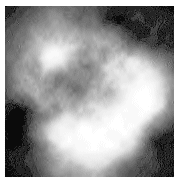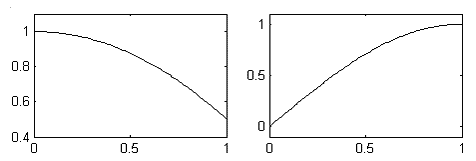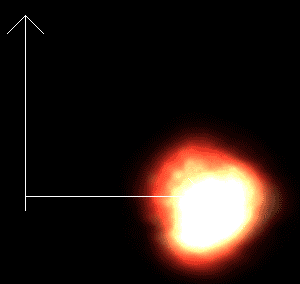# 3.12 创建一个3D爆炸效果，简单的粒子系统

## 解决方案## 工作原理

• 爆炸的初始位置的billboard中心点3D位置(用于billboarding) (Vector3 = 三个浮点数)
• 纹理坐标(用于billboarding) (Vector2 = 两个floats)
• 粒子创建的时间(一个浮点数)
• 粒子的存活时间(一个浮点数)
• 粒子移动的方向(Vector3 = 三个浮点数)
• 一个随机数，用于给每个粒子施加不同的行为(一个浮点数)

public struct VertexExplosion
{
public Vector3 Position;
public Vector4 TexCoord;

public VertexExplosion(Vector3 Position, Vector4 TexCoord, Vector4 AdditionalInfo)
{
this.Position = Position;
this.TexCoord = TexCoord;
}

public static readonly VertexElement[] VertexElements = new VertexElement[]
{
new VertexElement(0, 0, VertexElementFormat.Vector3, VertexElementMethod.Default, VertexElementUsage.Position, 0),
new VertexElement(0, 12, VertexElementFormat.Vector4, VertexElementMethod.Default, VertexElementUsage.TextureCoordinate, 0),
new VertexElement(0, 28, VertexElementFormat.Vector4, VertexElementMethod.Default, VertexElementUsage.TextureCoordinate, 1),
};

public static readonly int SizeInBytes = sizeof(float) * (3 + 4 + 4);
} 

Random rand;

rand = new Random();

private void CreateExplosionVertices(float time)
{
int particles = 80;
explosionVertices = new VertexExplosion[particles * 6];

int i = 0;
for (int partnr = 0; partnr < particles; partnr++)
{
Vector3 startingPos = new Vector3(5,0,0);
float r1 = (float)rand.NextDouble() - 0.5f;
float r2 = (float)rand.NextDouble() - 0.5f;
float r3 = (float)rand.NextDouble() - 0.5f;
Vector3 moveDirection = new Vector3(r1, r2, r3);
moveDirection.Normalize();

float r4 = (float)rand.NextDouble();
r4 = r4 / 4.0f * 3.0f + 0.25f;

explosionVertices[i++] = new VertexExplosion(startingPos, new Vector4(1, 1, time, 1000), new Vector4(moveDirection,r4));
explosionVertices[i++] = new VertexExplosion(startingPos, new Vector4(0, 0, time, 1000), new Vector4(moveDirection, r4));
explosionVertices[i++] = new VertexExplosion(startingPos, new Vector4(1, 0, time, 1000), new Vector4(moveDirection, r4));
explosionVertices[i++] = new VertexExplosion(startingPos, new Vector4(1, 1, time, 1000), new Vector4(moveDirection, r4));
explosionVertices[i++] = new VertexExplosion(startingPos, new Vector4(0, 1, time, 1000), new Vector4(moveDirection, r4));
explosionVertices[i++] = new VertexExplosion(startingPos, new Vector4(0, 0, time, 1000), new Vector4(moveDirection, r4));
}
} 

### HLSL

//XNA interface
float4x4 xView;
float4x4 xProjection;
float4x4 xWorld;
float3 xCamPos;
float3 xCamUp;
float xTime;

//Texture Samplers
Texture xExplosionTexture;
sampler textureSampler = sampler_state
{
texture = <xExplosionTexture>;
magfilter = LINEAR;
minfilter = LINEAR;
mipfilter=LINEAR;
};

struct ExpVertexToPixel
{
float4 Position : POSITION;
float2 TexCoord : TEXCOORD0;
float4 Color : COLOR0;
};

struct ExpPixelToFrame
{
float4 Color : COLOR0;
};

//Technique: Explosion
float3 BillboardVertex(float3 billboardCenter, float2 cornerID, float size)
{
float3 eyeVector = billboardCenter - xCamPos;
float3 sideVector = cross(eyeVector,xCamUp);
sideVector = normalize(sideVector);
float3 upVector = cross(sideVector,eyeVector);
upVector = normalize(upVector);
float3 finalPosition = billboardCenter;
finalPosition += (cornerID.x-0.5f)*sideVector*size;
finalPosition += (0.5f-cornerID.y)*upVector*size;

return finalPosition;
} 

ExpVertexToPixel ExplosionVS(float3 inPos: POSITION0, float4 inTexCoord: TEXCOORD0, float4 inExtra: TEXCOORD1)
{
ExpVertexToPixel Output = (ExpVertexToPixel)0;

float3 startingPosition = mul(inPos, xWorld);
float2 texCoords = inTexCoord.xy;
float birthTime = inTexCoord.z;
float maxAge = inTexCoord.w;
float3 moveDirection = inExtra.xyz;
float random = inExtra.w;
}

float age = xTime - birthTime; float relAge = age/maxAge;

float size = 1-relAge*relAge/2.0f;float sizer = saturate(1-relAge*relAge/2.0f);
float size = 5.0f*random*sizer; 

float totalDisplacement = sin(relAge*6.28f/4.0f);

float totalDisplacement = sin(relAge*6.28f/4.0f)*3.0f*random;

float3 billboardCenter = startingPosition + totalDisplacement*moveDirection;
billboardCenter += age*float3(0,-1,0)/1000.0f;

float3 finalPosition = BillboardVertex(billboardCenter, texCoords, size);
float4 finalPosition4 = float4(finalPosition, 1);
float4x4 preViewProjection = mul (xView, xProjection);
Output.Position = mul(finalPosition4, preViewProjection);

float alpha = 1-relAge*relAge;

Output.Color = float4(0.5f,0.5f,0.5f,alpha);

Output.TexCoord = texCoords;
return Output; 

ExpPixelToFrame ExplosionPS(ExpVertexToPixel PSIn) : COLOR0
{
ExpPixelToFrame Output = (ExpPixelToFrame)0;

Output.Color = tex2D(textureSampler, PSIn.TexCoord)*PSIn.Color;

return Output;
} 

technique Explosion
{
pass Pass0
{
}
} 

### 在XNA中设置Technique参数

expEffect.CurrentTechnique = expEffect.Techniques["Explosion"];
expEffect.Parameters["xWorld"].SetValue(Matrix.Identity);
expEffect.Parameters["xProjection"].SetValue(quatMousCam.ProjectionMatrix);
expEffect.Parameters["xView"].SetValue(quatMousCam.ViewMatrix);
expEffect.Parameters["xCamPos"].SetValue(quatMousCam.Position);
expEffect.Parameters["xExplosionTexture"].SetValue(myTexture);
expEffect.Parameters["xCamUp"].SetValue(quatMousCam.UpVector);
expEffect.Parameters["xTime"]. SetValue((float)gameTime.TotalGameTime.TotalMilliseconds); 

device.RenderState.AlphaBlendEnable = true;
device.RenderState.SourceBlend = Blend.SourceAlpha;
device.RenderState.DestinationBlend = Blend.One; 

finalColor=sourceBlend*sourceColor+destBlend*destColor

finalColor=sourceAlpha*sourceColor+1*destColor

1．找到存储在frame buffer中的像素的当前颜色，将三个颜色通道乘以1(对应前面规则中的1*destColor)。

3. 将两个颜色相加，将结果保存到frame buffer中。

### 关闭写入到Depth Buffer

• 每个粒子的每个像素都会与z-buffer中的当前内容(包含深度信息)作比较。如果在相机和爆炸之间已经有一个物体被绘制了，那么爆炸的粒子就通不过z-buffer测试，不会被绘制。
• 因为粒子不会改变z-buffer，就算第二个粒子在第一个粒子之后也会被绘制(当然，是在相机和爆炸间没有另一个物体的情况下)。

device.RenderState.DepthBufferWriteEnable = false;

expEffect.Begin();

foreach (EffectPass pass in expEffect.CurrentTechnique.Passes)
{
pass.Begin();
device.VertexDeclaration = new VertexDeclaration(device, VertexExplosion.VertexElements);
device.DrawUserPrimitives<VertexExplosion> (PrimitiveType.TriangleList, explosionVertices, 0, explosionVertices.Length / 3);
pass.End();
}
expEffect.End(); 

device.RenderState.DepthBufferWriteEnable = true;

if ((keyState.IsKeyDown(Keys.Space)))
CreateExplosionVertices((float) gameTime.TotalGameTime.TotalMilliseconds);

## 代码

private void CreateExplosionVertices(float time)
{
int particles = 80;
explosionVertices = new VertexExplosion[particles * 6];

int i = 0;
for (int partnr = 0; partnr < particles; partnr++)
{
Vector3 startingPos = new Vector3(5,0,0);
float r1 = (float)rand.NextDouble() - 0.5f;
float r2 = (float)rand.NextDouble() - 0.5f;
float r3 = (float)rand.NextDouble() - 0.5f;
Vector3 moveDirection = new Vector3(r1, r2, r3);
moveDirection.Normalize();

float r4 = (float)rand.NextDouble();
r4 = r4 / 4.0f * 3.0f + 0.25f;

explosionVertices[i++] = new VertexExplosion(startingPos, new Vector4(1, 1, time, 1000), new Vector4(moveDirection, r4));
explosionVertices[i++] = new VertexExplosion(startingPos, new Vector4(0, 0, time, 1000), new Vector4(moveDirection, r4));
explosionVertices[i++] = new VertexExplosion(startingPos, new Vector4(1, 0, time, 1000), new Vector4(moveDirection, r4));
explosionVertices[i++] = new VertexExplosion(startingPos, new Vector4(1, 1, time, 1000), new Vector4(moveDirection, r4));
explosionVertices[i++] = new VertexExplosion(startingPos, new Vector4(0, 1, time, 1000), new Vector4(moveDirection, r4));
explosionVertices[i++] = new VertexExplosion(startingPos, new Vector4(0, 0, time, 1000), new Vector4(moveDirection, r4));
}
} 

protected override void Draw(GameTime gameTime)
{
device.Clear(ClearOptions.Target | ClearOptions.DepthBuffer, Color.Black, 1, 0);
cCross.Draw(quatCam.ViewMatrix, quatCam.ProjectionMatrix);

if (explosionVertices != null)
{
//draw billboards
expEffect.CurrentTechnique = expEffect.Techniques["Explosion"];
expEffect.Parameters["xWorld"].SetValue(Matrix.Identity);
expEffect.Parameters["xProjection"].SetValue(quatCam.ProjectionMatrix);
expEffect.Parameters["xView"].SetValue(quatCam.ViewMatrix);
expEffect.Parameters["xCamPos"].SetValue(quatCam.Position);
expEffect.Parameters["xExplosionTexture"].SetValue(myTexture);
expEffect.Parameters["xCamUp"].SetValue(quatCam.UpVector);
expEffect.Parameters["xTime"]. SetValue((float)gameTime.TotalGameTime.TotalMilliseconds);

device.RenderState.AlphaBlendEnable = true;
device.RenderState.SourceBlend = Blend.SourceAlpha;
device.RenderState.DestinationBlend = Blend.One;
device.RenderState.DepthBufferWriteEnable = false;

expEffect.Begin();
foreach (EffectPass pass in expEffect.CurrentTechnique.Passes)
{
pass.Begin();
device.VertexDeclaration = new VertexDeclaration(device, VertexExplosion.VertexElements);
device.DrawUserPrimitives<VertexExplosion> (PrimitiveType.TriangleList, explosionVertices, 0, explosionVertices.Length / 3);
pass.End();
}
expEffect.End();

device.RenderState.DepthBufferWriteEnable = true;
}
base.Draw(gameTime);
} 

ExpVertexToPixel ExplosionVS(float3 inPos: POSITION0, float4 inTexCoord: TEXCOORD0, float4 inExtra: TEXCOORD1)
{
ExpVertexToPixel Output = (ExpVertexToPixel)0;

float3 startingPosition = mul(inPos, xWorld);
float2 texCoords = inTexCoord.xy;
float birthTime = inTexCoord.z;
float maxAge = inTexCoord.w;
float3 moveDirection = inExtra.xyz;
float random = inExtra.w;

float age = xTime - birthTime;
float relAge = age/maxAge;
float sizer = saturate(1-relAge*relAge/2.0f);
float size = 5.0f*random*sizer;

float totalDisplacement = sin(relAge*6.28f/4.0f)*3.0f*random;
float3 billboardCenter = startingPosition + totalDisplacement*moveDirection;
billboardCenter += age*float3(0,-1,0)/1000.0f;
float3 finalPosition = BillboardVertex(billboardCenter, texCoords, size);
float4 finalPosition4 = float4(finalPosition, 1);

float4x4 preViewProjection = mul (xView, xProjection);
Output.Position = mul(finalPosition4, preViewProjection);

float alpha = 1-relAge*relAge;
Output.Color = float4(0.5f,0.5f,0.5f,alpha);

Output.TexCoord = texCoords;

return Output;
}